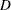The AUTOREG Procedure
 PROC AUTOREG Statement
PROC AUTOREG options ;

The following options can be used in the PROC AUTOREG statement:

DATA=SAS-data-set

specifies the input SAS data set. If the DATA= option is not specified, PROC AUTOREG uses the most recently created SAS data set.

OUTEST=SAS-data-set

writes the parameter estimates to an output data set. See the section OUTEST= Data Set later in this chapter for information on the contents of these data set.

COVOUT

writes the covariance matrix for the parameter estimates to the OUTEST= data set. This option is valid only if the OUTEST= option is specified.

PLOTS<(global-plot-options)> < = (specific plot options)>

requests that the AUTOREG procedure produce statistical graphics via the Output Delivery System, provided that the ODS GRAPHICS statement has been specified. For general information about ODS Graphics, see Chapter 21, Statistical Graphics Using ODS (SAS/STAT 9.22 User's Guide). The global-plot-options apply to all relevant plots generated by the AUTOREG procedure. The global-plot-options supported by the AUTOREG procedure follow.

### Global Plot Options

ONLY

suppresses the default plots. Only the plots specifically requested are produced.

UNPACKPANEL

breaks a graphic that is otherwise paneled into individual component plots.

### Specific Plot Options

ALL

requests that all plots appropriate for the particular analysis be produced.

ACF

produces the autocorrelation function plot.

IACF

produces the inverse autocorrelation function plot of residuals.

PACF

produces the partial autocorrelation function plot of residuals.

FITPLOT

plots the predicted and actual values.

COOKSD

produces the Cook’splot.

QQ

Q-Q plot of residuals.

RESIDUAL | RES

plots the residuals.

STUDENTRESIDUAL

plots the studentized residuals. For the models with the NLAG= or GARCH= options in the MODEL statement or with the HETERO statement, this option is replaced by the STANDARDRESIDUAL option.

STANDARDRESIDUAL

plots the standardized residuals.

WHITENOISE

plots the white noise probabilities.

RESIDUALHISTOGRAM | RESIDHISTOGRAM

plots the histogram of residuals.

NONE

suppresses all plots.

In addition, any of the following MODEL statement options can be specified in the PROC AUTOREG statement, which is equivalent to specifying the option for every MODEL statement: ALL, ARCHTEST, BACKSTEP, CENTER, COEF, CONVERGE=, CORRB, COVB, DW=, DWPROB, GINV, ITER, ITPRINT, MAXITER=, METHOD=, NOINT, NOMISS, NOPRINT, and PARTIAL.Previous Page | Next Page | Top of Page